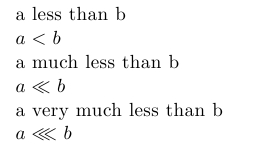less than sign(s)

# LaTeX less than signs

StartLaTeX faqLaTeX topicsLaTeX course
< footnote within a table     > greater than

## less than

If a is less than b you could wrote a < b just use the normal char < ; if a is much less than b then a « b where « would be the command \ll; and finally if a is very small compared with b then you could use the \lll command which requires usepackage amssymb.

### example

\documentclass{article}
\begin{document}
\ \\
a less than b \\
$a < b$ \\
a much less than b \\
$a \ll b$ \\
\end{document}

Output:### example very much less than

\documentclass{article}
\usepackage{amssymb}
\begin{document}
\ \\
a less than b \\
$a < b$ \\
a much less than b \\
$a \ll b$ \\
a very much less than b \\
$a \lll b$\\
\end{document}Contact